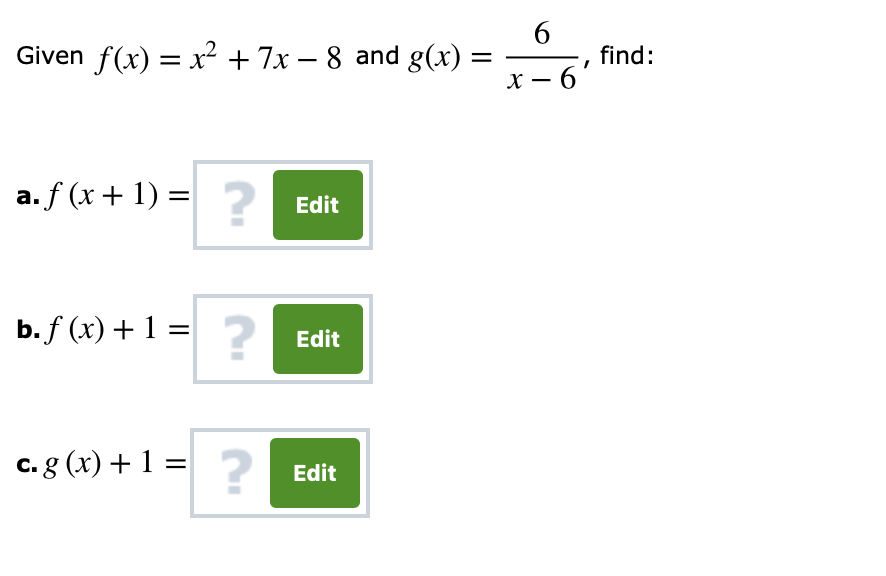# Given f (x) = x2 + 7-8 and g(x) =-, find: x-6 2 a,f(x + 1) = Edit b,f (x) + 1 Edit Edit

Questionhelp_outlineImage TranscriptioncloseGiven f (x) = x2 + 7-8 and g(x) =-, find: x-6 2 a,f(x + 1) = Edit b,f (x) + 1 Edit Edit fullscreen

### Want to see this answer and more?

Experts are waiting 24/7 to provide step-by-step solutions in as fast as 30 minutes!*